# Kg to lbs in Excel

1 kg = 2.20462 lbs (pounds) and 1 lb (pound) = 0.45359 kg. Use a simple formula, the CONVERT function or download our free unit converter to convert from kg to lbs or vice versa (see below).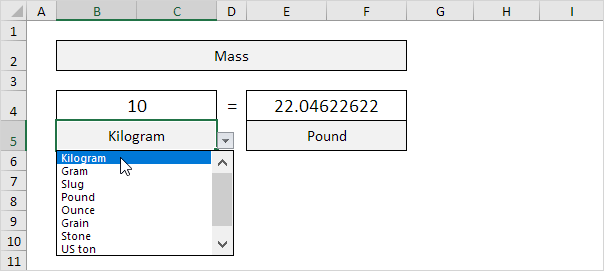1. First, to convert from kg to lbs, multiply by 2.20462. The simple formula below does the trick.2. To convert from lbs to kg, divide by 2.20462.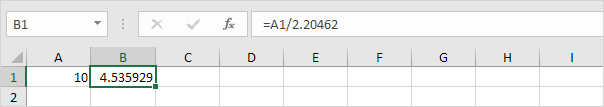3. You can also use the CONVERT function in Excel to convert from kg to lbs.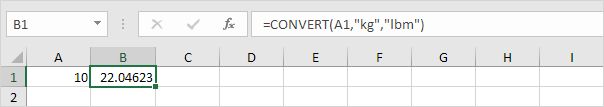Note: the CONVERT function has three arguments (number, from_unit and to_unit). The unit abbreviation lbm stands for pound mass.

4. You can also use the CONVERT function in Excel to convert from lbs to kg.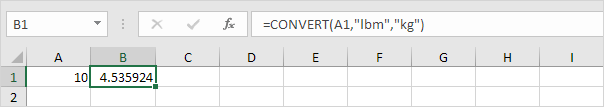Download our free unit converter to quickly and easily convert from kg to lbs (or vice versa).

5. Select Mass from the category drop-down list.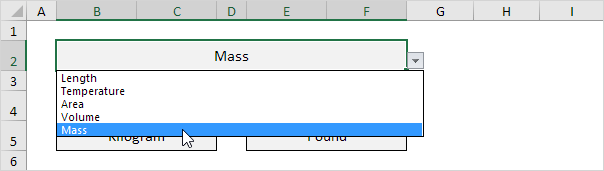Explanation: because you selected Mass from this drop-down list, the dependent drop-down lists in cell B5 and cell E5 now contain mass units.

6. Enter a number and select the correct mass units.Explanation: the unit converter uses worksheet change events to automatically execute VBA code when you change a cell (B2, B4, B5, E4 or E5). The VBA code uses the CONVERT function to convert from one measurement unit to another and uses the VLOOKUP function to lookup the correct unit abbreviations (kg, g, lbm, ozm, etc.) stored on the second worksheet.

Go to Next Chapter: Keyboard Shortcuts